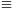Mathematical and Physical Journal
for High Schools
Issued by the MATFUND Foundation
 Already signed up? New to KöMaL?

#Problem A. 393. (February 2006)

A. 393. Prove that if n>1 is an integer such that 3n+4n is divisible by n then n is divisible by 7.

(5 pont)

Deadline expired on March 16, 2006.

The number 3n+4n is odd and not divisible by 3, therefore n is odd as well and not divisible by 3.

Write n=2k+1. Then

3n+4n=3.9k+42k+13.(-1)k-1 (mod 5).

This number is not divisible by 5 for any value of n, so neither 5 is a divisor of n.

Let p be the smallest prime divisor of n and c is that residue class for which 4c3 (mod p). Then

4n(c2n-1)=(cn-1)((4c)n+4n)(cn-1)(3n+4n)0 (mod p)

and

 (1) c2n1 (mod p).

By Fermat's theorem,

 (2) cp-11!(mod p).

Let d be the order of c modulo p. By (1) and (2), d is a common divisor of 2n and p-1, so d is a divisor of their GCD which is 2. Therefore d|2 and

p|c2-1=(c+1)(c-1).

If p|c-1 then 44c3 (mod p) which is a contradiction. So we have p|c+1 and

04(c+1)=4c+43+4=7 (mod p).

This implies p=7.

### Statistics:

 10 students sent a solution. 5 points: Erdélyi Márton, Estélyi István, Fischer Richárd, Gyenizse Gergő, Jankó Zsuzsanna, Kisfaludi-Bak Sándor, Nagy 224 Csaba, Paulin Roland, Tomon István. 1 point: 1 student.

Problems in Mathematics of KöMaL, February 2006